Next: B.2 Noise and Distortion Up: B. Sigma Delta Converters Previous: B. Sigma Delta Converters

# B.1 First and Second-Order Noise Shaping Loops

The basic principle of a first-order noise shaping sigma-delta ADC is shown in Fig. B.1. The input signal x(t) is fed through an integrator and is then quantized, usually with only one bit resolution. The quantizer output is then sampled at a high frequency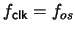and subtracted from the input. Thus, average of the digital output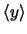equals the input x(t). As a consequence of the oversampling and of the integration most of the noise contained in the digital output y lies outside of the signal band and is eliminated by a subsequent digital low-pass filter (usually a decimation filter to reduce the sampling frequency from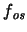to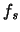) which is not shown in Fig. B.1.

An improvement of the signal-to-noise (SNR) ratio can be achieved by increasing the order of the integrating filter as shown in Fig. B.2. The resulting SNR at full-range input is given by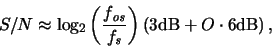(B.1)

where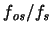is the oversampling rate, which spreads the noise over a wider band, and O is the order, which determines the noise shaping. The drawback of the increased order is a reduced input signal range, which somewhat reduces the achievable SNR.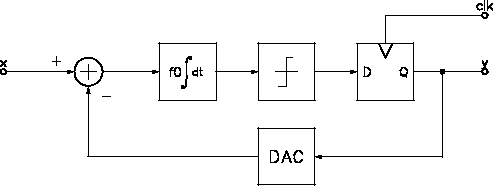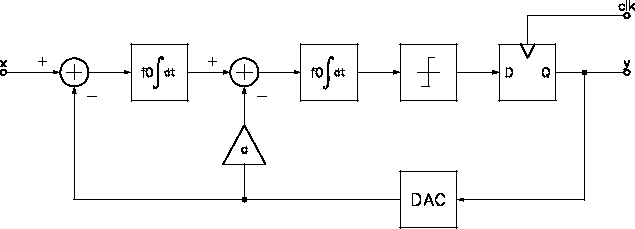Next: B.2 Noise and Distortion Up: B. Sigma Delta Converters Previous: B. Sigma Delta Converters

G. Schrom